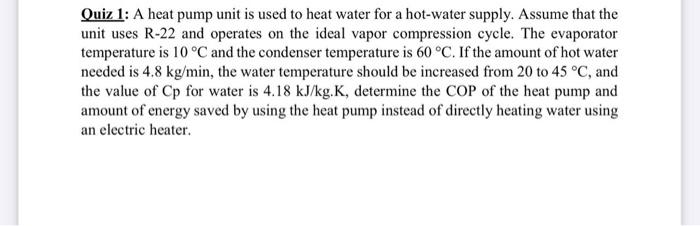# Question Solved1 AnswerQuiz 1: A heat pump unit is used to heat water for a hot-water supply. Assume that the unit uses R-22 and operates on the ideal vapor compression cycle. The evaporator temperature is 10 °C and the condenser temperature is 60 °C. If the amount of hot water needed is 4.8 kg/min, the water temperature should be increased from 20 to 45 °C, and the value of Cp for water is 4.18 kJ/kg.K, determine the COP of the heat pump and amount of energy saved by using the heat pump instead of directly heating water using an electric heater.1MNT90 The Asker · Mechanical EngineeringTranscribed Image Text: Quiz 1: A heat pump unit is used to heat water for a hot-water supply. Assume that the unit uses R-22 and operates on the ideal vapor compression cycle. The evaporator temperature is 10 °C and the condenser temperature is 60 °C. If the amount of hot water needed is 4.8 kg/min, the water temperature should be increased from 20 to 45 °C, and the value of Cp for water is 4.18 kJ/kg.K, determine the COP of the heat pump and amount of energy saved by using the heat pump instead of directly heating water using an electric heater.
More
Transcribed Image Text: Quiz 1: A heat pump unit is used to heat water for a hot-water supply. Assume that the unit uses R-22 and operates on the ideal vapor compression cycle. The evaporator temperature is 10 °C and the condenser temperature is 60 °C. If the amount of hot water needed is 4.8 kg/min, the water temperature should be increased from 20 to 45 °C, and the value of Cp for water is 4.18 kJ/kg.K, determine the COP of the heat pump and amount of energy saved by using the heat pump instead of directly heating water using an electric heater.